Processing ......FreeComputerBooks.com Free Computer, Mathematics, Technical Books and Lecture Notes, etc.

Computational Simulations and Modeling
Related Book Categories:
•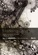Modeling Creativity - Case Studies in Python (Tom De Smedt)

This book is to model creativity using computational approaches in Python. The aim is to construct computer models that exhibit creativity in an artistic context, that is, that are capable of generating or evaluating an artwork (visual or linguistic), etc.

•Modeling and Simulation in Python (Allen B. Downey)

This book is an introduction to physical modeling using a computational approach with Python. You will learn how to use Python to accomplish many common scientific computing tasks: importing, exporting, and visualizing data; numerical analysis; etc.

•Computer Simulation Techniques - The Definitive Introduction

This book addresses all the important aspects of a computer simulation study, including modeling, simulation languages, validation, input probability distribution, and analysis of simulation output data.

•MATLAB - Modelling, Programming and Simulations (E. P. Leite)

This is an authoritative guide to generating readable, compact, and verifiably correct MATLAB programs. It is ideal for undergraduate engineering courses in Mechanical, Aeronautical, Civil, and Electrical engineering that require/use MATLAB.

•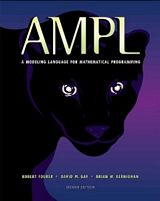AMPL: A Modeling Language for Mathematical Programming

This book is a complete guide to AMPL for modelers at all levels of experience. It begins with a tutorial on widely used linear programming models, and presents all of AMPL's features for linear programming with extensive examples.

•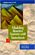Modeling Reactive Systems With Statecharts (David Harel)

The book provides a detailed description of a set of languages for modelling reactive systems, which underlies the STATEMATE toolset. The approach is dominated by the language of Statecharts, used to describe behavior and activities.

•The Nature of Code: Simulating Natural Systems with Processing

A range of programming strategies and techniques behind computer simulations of natural systems, from elementary concepts in mathematics and physics to more advanced algorithms that enable sophisticated visual results, using Processing.

•Computational and Numerical Simulations (Jan Awrejcewicz)

It presents both new theories and their applications, showing bridge between theoretical investigations and possibility to apply them by engineers of different branches of science.

•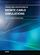Theory and Applications of Monte Carlo Simulations (Wai Kin Chan)

The purpose of this book is to introduce researchers and practitioners to recent advances and applications of Monte Carlo Simulation (MCS). Random sampling is the key of the MCS technique.

•Applications of Monte Carlo Method in Science and Engineering

This book exposes the broad range of applications of Monte Carlo simulation in the fields of Quantum Physics, Statistical Physics, Reliability, Medical Physics, Polycrystalline Materials, Ising Model, Chemistry, Agriculture, Food Processing, X-ray Imaging, ...

•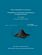From Algorithms to Z-Scores: Probabilistic and Statistical Modeling

This is a textbook for a course in mathematical probability and statistics for computer science students.

•Technology and Engineering Applications of SIMULINK

Present the procedural steps required for modeling and simulating the basic dynamic system problems in SIMULINK, built on the top of MATLAB to provide a platform for engineers to plan, model, design, simulate, test and implement complex systems.

•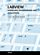Modeling, Programming and Simulations Using LabVIEW™ Software

In this book a collection of applications covering a wide range of possibilities is presented. We go from simple or distributed control software to modeling done in LabVIEW to very specific applications to usage in the educational environment.

•Learning Processing: Guide to Programming Images, Animation

This book teaches you the basic building blocks of programming needed to create cutting-edge graphics applications including interactive art, live video processing, and data visualization, using Processing..

•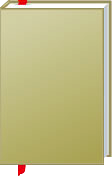Computational Simulations and Modeling

This is the previous page of Computational Simulations and Modeling, we are in the processing to convert all the books there to the new page. Please check this page again!!!

Book CategoriesAll CategoriesRecent BooksIT Research LibraryMiscellaneous Books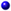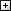Computer LanguagesComputer ScienceData Science/DatabasesElectronic EngineeringJava and Java EE (J2EE)Linux and UnixMathematicsMicrosoft and .NETMobile ComputingNetworking and CommunicationsSoftware EngineeringSpecial TopicsWeb Programming
Other Categories